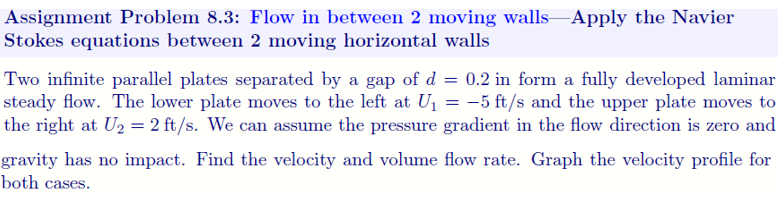Home / Answered Questions / Other / assignment-problem-8-3-flow-in-between-2-moving-walls-apply-the-navier-stokes-equations-between-2-mo-aw780

# (Solved): Assignment Problem 8.3: Flow In Between 2 Moving Walls-Apply The Navier Stokes Equations Between 2 M...Assignment Problem 8.3: Flow in between 2 moving walls-Apply the Navier Stokes equations between 2 moving horizontal walls Two infinite parallel plates separated by a gap of d = 0.2 in form a fully developed laminar steady flow. The lower plate moves to the left at U1 = -5 ft/s and the upper plate moves to the right at U2 = 2 ft/s. We can assume the pressure gradient in the flow direction is zero and gravity has no impact. Find the velocity and volume flow rate. Graph the velocity profile for both cases.

We have an Answer from Expert